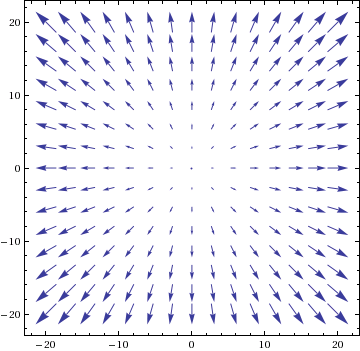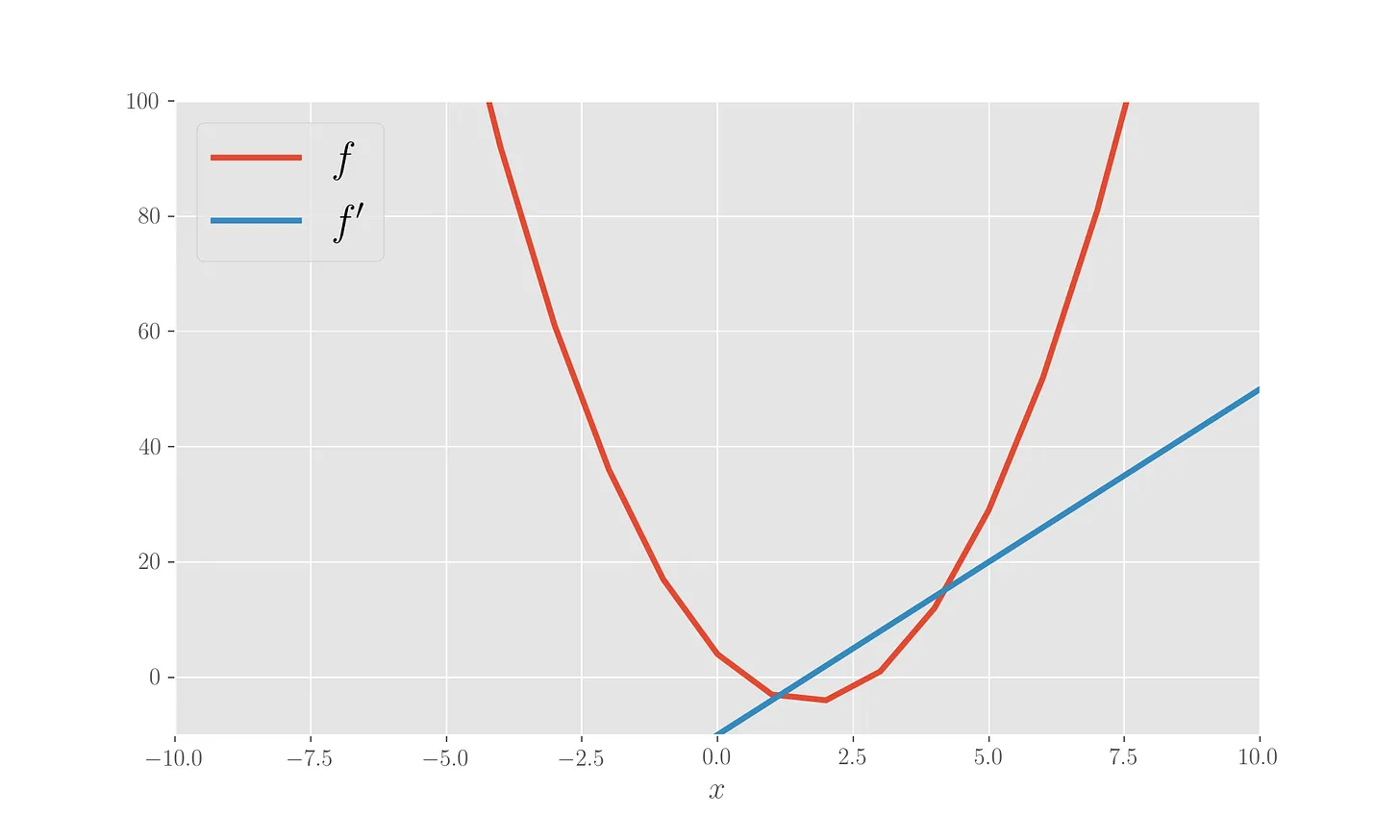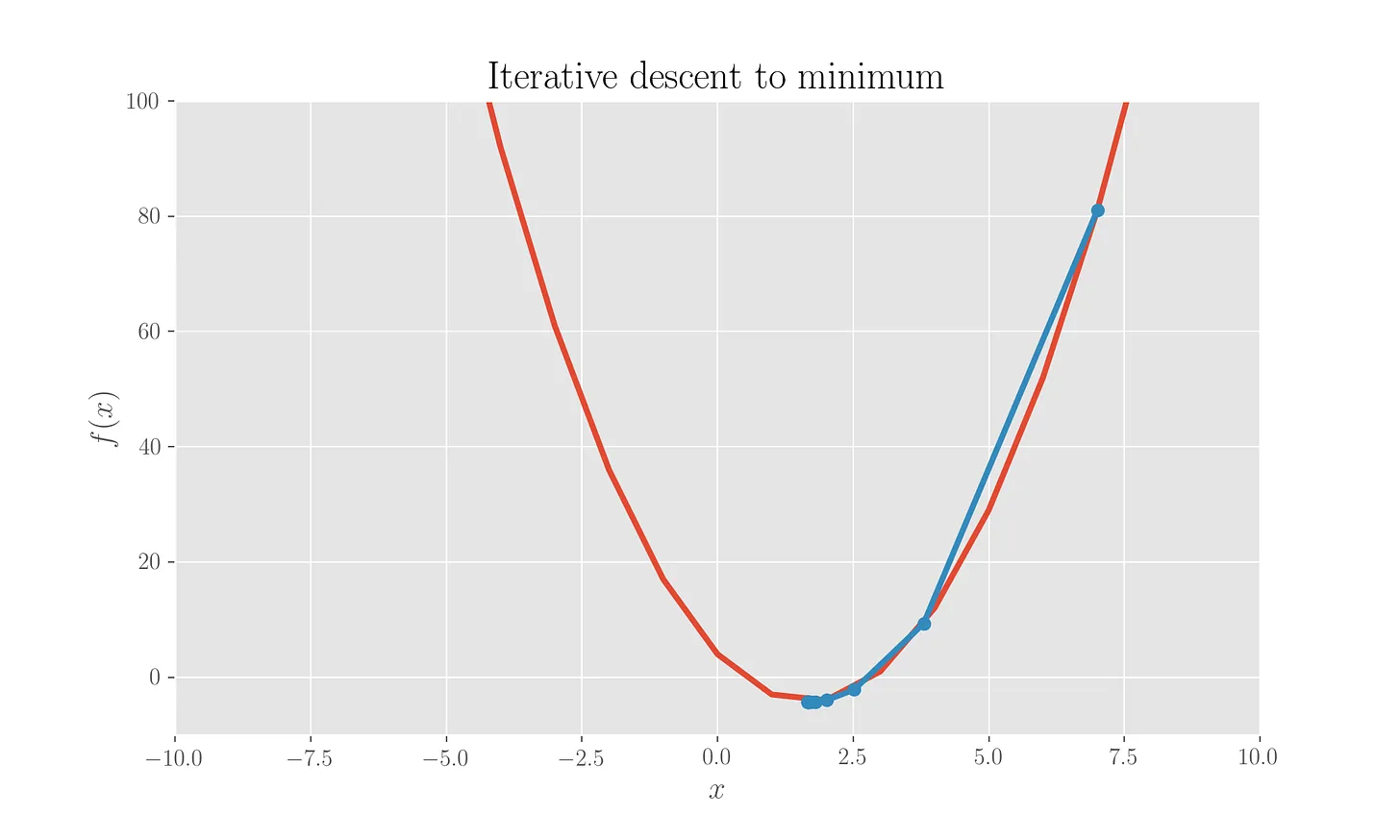# Dissecting the Gradient Descent method

A full Jupyter notebook outlining what is done in this post is reachable here.

Gradient Descent is a mathematical optimization method used to minimize functions in the form of a sum:

$f(w) = \sum_i^n f_i(w)$

This method lies at the core of the mathematical tools used in Machine Learning. For instance, in a Linear Regression task, in the Ordinary Least Squares implementation, the function to be minimized (error function) is the one describing the sum of squared residuals between the observed value and the value predicted by the fitting line, over all observations:

$E[\bar w] = \sum_i^n (y_i - \bar w \cdot x_i)^2 \ .$

Here (sum goes over all observations in the dataset), w is the vector of parameters of the line, the dot product is intended to assume a value of 1 for observational independent variable x, so that we would have the slope and the intercept of the line as parameters, in the parameters vector; y is the actual observed dependent variable. The Gradient Descent method is a first order optimization method in that it deals with the first derivative of the function to be minimized. The gradient of the function is indeed used to identify the direction of maximum growth of the function, hence a descent is implemented, whereby the parameters get, starting from an initial state, diminished following the updating rule:

$w := w - \alpha \nabla f(w) = w - \alpha \nabla \left( \sum_i^n f_i(w) \right) \ .$

At each iteration step, a Standard Gradient Descent needs to update the parameters by computing the gradient considering all training points in the dataset. The value multiplying the gradient is the learning rate chosen to perform the update. Clearly, a too big learning rate might get us past the minumum we want to reach, trapping us in a zig-zagging path across it. On the other hand, a value of the learning rate which is very small would slow down the computation. The iteration will stop once the difference between the current value of the parameter and the previous one falls below a given precision threshold.

## Standard Gradient Descent: finding the minumum of a function

Suppose we want to use the (standard) Gradient Descent method to minimize a function. Given a paraboloid

$f(x, y) = x^2 + y^2 \ ,$

the vector field given by its gradient is graphically represented as per figure (vector lengths are scaled).Obviously we know, from calculus, that the (global) minimum will be in the origin. The gradient vector field is furnishing the direction of maximum growth of the paraboloid, hence a descent (a negative sign in the parameter update) will lead us towards the desired minimum.The second graph here shows a Gradient Descent towards the minumum of a 1D parabola from a starting point $$x = 7$$ and using a learning rate equal to $$10^{-1}$$ .

The computation has been stopped when the difference between the iteration updates was below a threshold value for the precision set to $$10^{-4}$$.

## Standard Gradient Descent: implementing a Linear Regression

As we said, this method is used in a Ordinary Least Squares calculation in a Linear Regression to find the line which best fits a series of observation points. We will now use Python to “manually” compute a Linear Regression through Gradient Descent. We need Numpy and (but we could calculate it ourselves) the euclidean distance method in Scipy:

import numpy as numpy
from scipy.spatial.distance import euclidean
Suppose we have the experimental points
x = np.array([1, 2, 2.5, 3, 3.5, 4.5, 4.7, 5.2, 6.1, 6.1, 6.8])
y = np.array([1.5, 1, 2, 2, 3.7, 3, 5, 4, 5.8, 5, 5.7])


and setting the learning rate and the precision at

alpha = 0.001                       # learning rate
p = 0.001


we can define the methods

def f(x, w):
"""A line y = wx, to be intended as w0 + w1x (x0 = 1)"""
return np.dot(x, w)

def diff(a, b):
return a - b

def squared_diff(a, b):
return (a - b)**2

def obj_f(w, x, y):
"""The objective function: sum of squared diffs between observations
and line predictions"""
return sum([squared_diff(f(np.array([1, x[i]]), w), y[i])
for i in range(len(x))])

def obj_f_der(w, x, y):
"""Gradient of the objective function in the parameters"""
return sum([np.dot(2 * np.array([1, x[i]]),
diff(f(np.array([1, x[i]]), w), y[i]))
for i in range(len(x))])


Now, all we need to do in order to implement a Gradient Descent is

former_w = np.array([10, 5])    # the chosen starting point for the descent
while True:

w = former_w - alpha * obj_f_der(former_w, x, y)

if euclidean(former_w, w) <= p:
break
else:
former_w = w


The result of the Gradient Descent is an intercept $$0.27$$ and a slope $$0.80$$ for the fitting line, which is shown in the following figure along with the experimental points.## Stochastic Gradient Descent: reimplementation of the Linear Regression

The stochastic version of the Gradient Descent method does not use all points at each iteration to calculate the gradient of the function but rather picks one point, randomly extracted from the dataset, to compute said gradient. The update of the parameters will then be, at each iteration,

$w := w - \alpha \nabla f_i(w)$

A for loop will scroll this rule across all observations in the training set , which has been randomly shuffled (epoch), until the updated parameter does not change by more than chosen precision with respect to the previous one. The Stochastic Gradient Descent will be particularly useful for large datasets where the standard updating rule might be too slow to compute. Using the same dataset, same learning rate and same precision as before, a Stochastic Gradient Descent leads to intercept equal to $$0.21$$ and slope equal to $$0.81$$, shown in figure.Tags:

Categories:

Created: